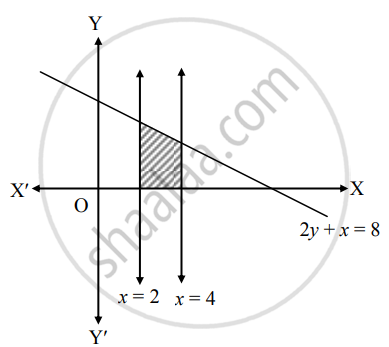# Using integration, find the area of the region bounded by the line 2y + x = 8 , X−axis and the lines x = 2 and x = 4 - Mathematics and Statistics

Sum

Using integration, find the area of the region bounded by the line 2y + x = 8 , X−axis and the lines x = 2 and x = 4

#### Solution

Let A be the required area.

Consider the equation 2y + x = 8.

∴ y = 1/2(8 - x)∴ Required area = int_2^4 y  "d"x

= int_2^4 1/2(8 - x) "d"x

= 1/2[8x - x^2/2]_2^4

= 1/2[(8(4) - 4^2/2) - (8(2) - ^2/2)]

= 1/2[(32 - 8) - (16 - 2)]

= 5 sq.units

Concept: Area Bounded by the Curve, Axis and Line
Is there an error in this question or solution?